# UniRE: A Unified Label Space for Entity Relation Extraction

## UniRE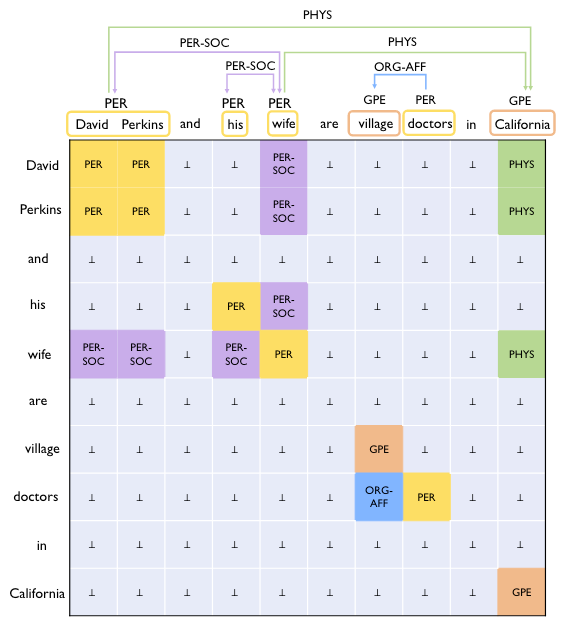### Biaffine Model

$$\left\{\mathbf{h}_1, \ldots, \mathbf{h}_{|s|}\right\}=\operatorname{PLM}\left(\left\{\mathbf{x}_1, \ldots, \mathbf{x}_{|s|}\right\}\right)$$

$\mathbf{h_i}$ 为文中每个词WordPiece后的首个Token的表示, 代替了每个词的表示作为Entity的分割边界, 这样可以减少不必要的输入和计算.

\begin{aligned} \mathbf{h}_i^{\text {head }}&=\operatorname{MLP}_{\text {head }}\left(\mathbf{h}_i\right) \\ \quad \mathbf{h}_i^{\text {tail }}&=\operatorname{MLP}_{\text {tail }}\left(\mathbf{h}_i\right) \end{aligned}

\begin{aligned} \mathbf{g}_{i, j} &=\operatorname{Biaff}\left(\mathbf{h}_i^{\text {head }}, \mathbf{h}_j^{\text {tail }}\right) \\ \operatorname{Biaff}\left(\mathbf{h}_1, \mathbf{h}_{\mathbf{2}}\right) &=\mathbf{h}_1^T \mathbf{U}_1 \mathbf{h}_2+\mathbf{U}_2\left(\mathbf{h}_1 \oplus \mathbf{h}_2\right)+\mathbf{b} \end{aligned}

class Biaffine(nn.Module):
def __init__(self, in_size, out_size, bias_x=True, bias_y=True):
super().__init__()
self.in_size = in_size
self.out_size = out_size
self.bias_x = bias_x
self.bias_y = bias_y

weight = torch.zeros((in_size + int(bias_x), out_size, in_size + int(bias_y)))
nn.init.xavier_normal_(weight)
self.weight = nn.Parameter(weight)

def forward(self, x, y):
if self.bias_x:
x = torch.cat([x, torch.ones_like(x[..., :1])], dim=-1)
if self.bias_y:
y = torch.cat((y, torch.ones_like(y[..., :1])), dim=-1)
hidden = torch.einsum("bxi,ioj,byj->bxyo", x, self.weight, y)
return hidden

### Table Filling

$$P\left(\mathbf{y}_{i, j} \mid s\right)=\operatorname{Softmax}\left(\mathrm{dropout}\left(\mathbf{g}_{i, j}\right)\right)$$

$$\mathcal{L}_{\text {entry }}=-\frac{1}{|s|^2} \sum_{i=1}^{|s|} \sum_{j=1}^{|s|} \log P\left(\mathbf{y}_{i, j}=y_{i, j} \mid s\right)$$

### Constraints

#### Symmetry

$$\mathcal{L}_{\mathrm{sym}}=\frac{1}{|s|^{2}} \sum_{i=1}^{|s|} \sum_{j=1}^{|s|} \sum_{t \in \mathcal{Y}_{\mathrm{sym}}}\mid \mathcal{P}_{i, j, t}-\mathcal{P}_{j, i, t}\mid$$

$\mathcal{Y}_\text{sym}$ 代表对称的标签, 也就是实体类型标签和对称关系类型的集合.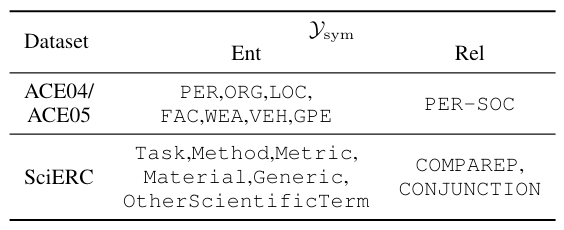#### Implication

$$\mathcal{L}_{\mathrm{imp}}=\frac{1}{|s|} \sum_{i=1}^{|s|}\left[\max _{l \in \mathcal{Y}_r}\left\{\mathcal{P}_{i,:, l}, \mathcal{P}_{:, i, l}\right\}-\max _{t \in \mathcal{Y}_e}\left\{\mathcal{P}_{i, i, t}\right\}\right]_\ast$$

Hinge Loss可以最大化这两类概率之间的差值, 也就是让关系的概率更小些, 实体类型的概率更大些.

$$\mathcal{L}=\mathcal{L}_{\text {entry }}+\mathcal{L}_{\text {sym }}+\mathcal{L}_{\mathrm{imp}}$$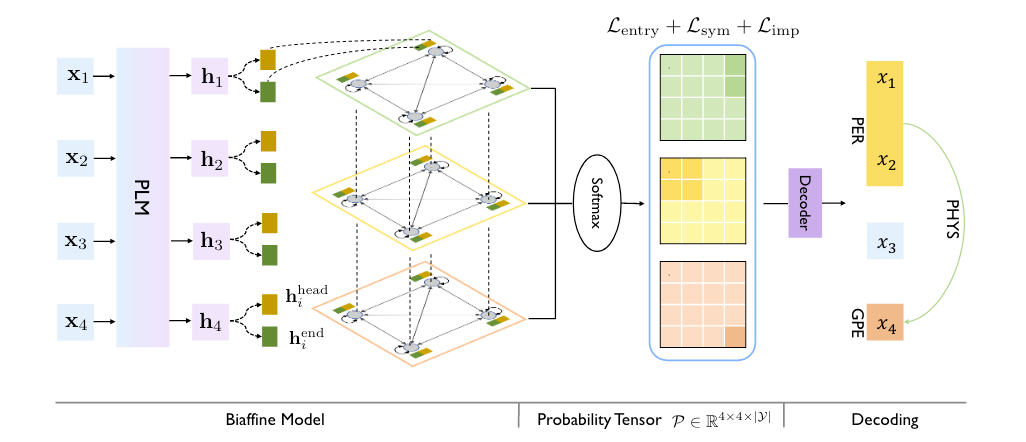### Decoding

1. 解码Span以识别实体边界.
2. 解码实体的实体类型.
3. 解码实体对的关系类型.

#### Span Decoding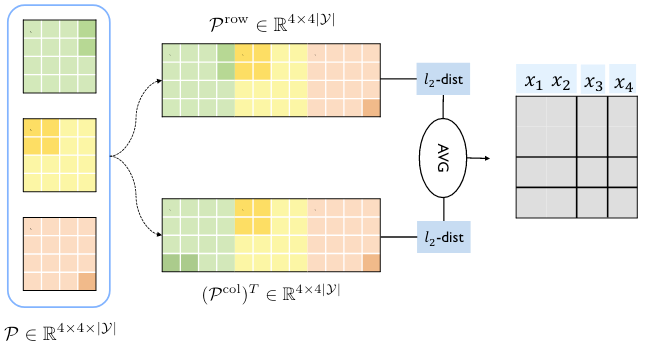#### Entity Type Decoding

$$\hat{t}=\arg \max _{t \in \mathcal{Y}_e \cup\{\perp\}} \operatorname{Avg}\left(\mathcal{P}_{i: j, i: j, t}\right)$$

#### Relation Type Decoding

$$\hat{l}=\arg \max _{l \in \mathcal{Y}_r \cup\{\perp\}} \operatorname{Avg}\left(\mathcal{P}_{i: j, m: n, l}\right)$$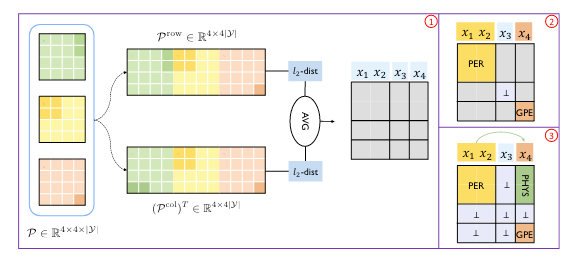## Experiments

### Datasets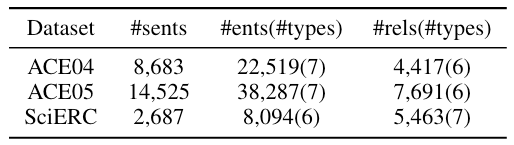### Performance Comparison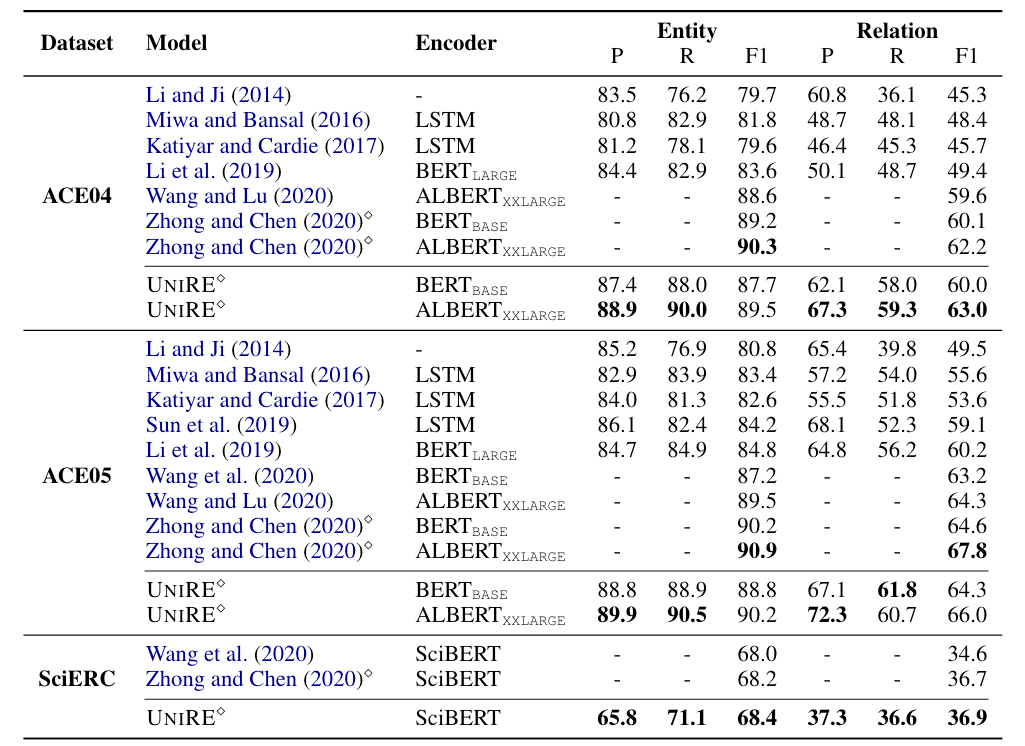Wang et al.和Zhong and Chen分别对应着20年ERE方法TSEPURE. UniRE在ACE05上的表现并没有PURE好, 但在更困难的SciERC上效果略好于PURE. 在ACE04上的表现比Baseline好得多.

### Ablation Study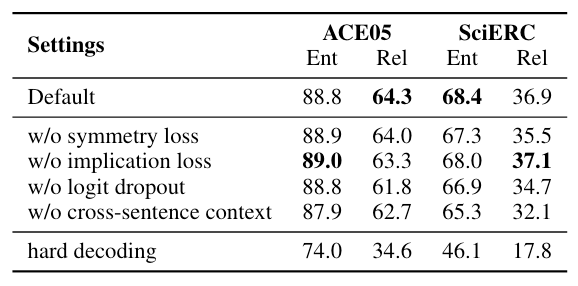• Symmetry Loss: 在ACE05上实体识别稍微掉了一点, 但是在SciERC上有明显涨点. 它对实体分类有效, 在两个数据集上似乎对关系分类也很有帮助的.
• Implication Loss: 在ACE05上移除会有害于关系预测, 发挥了不小作用, SciERC上则是稍稍一点副作用.
• Logit Dropout: 在两个数据集上, 使用Logit Dropout带来了两个点的提升.
• Cross - Sentence Context: 像PURE里面一样, 也使用一个跨句的窗口来捕捉长距离依赖. 使用更大的上下文有益于任务.
• Hard Decoding: 直接使用概率分布硬解码, 而不使用作者提出的解码算法. 对于正方形 / 长方形, 对应的实体类型 / 关系类型均取最高频次者. 如果直接硬解码, 效果会非常差, 证明了作者的解码方法有效.

### Inference Speed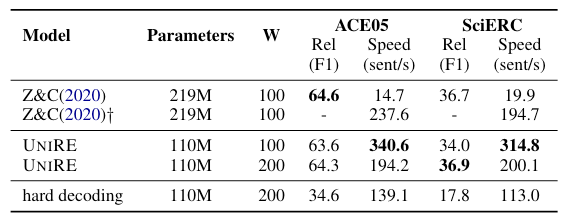PURE因为采用了两个独立的Encoder所以参数量是UniRE的两倍. 从结果中可以看出, UniRE比PURE的近似模型推理速度快不少. 性能上不相上下.

### Impact of Different Threshold α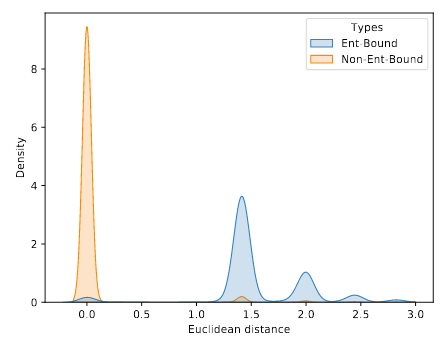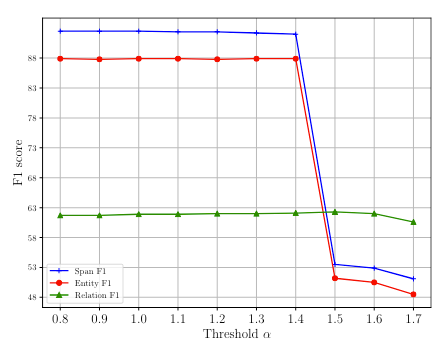### Context Window and Logit Dropout Rate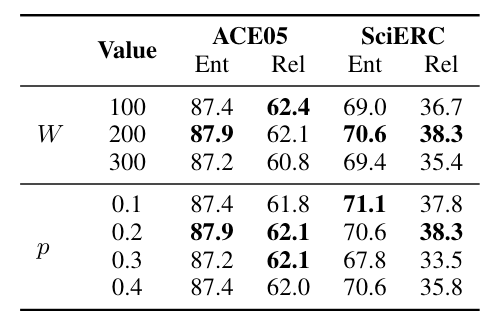Logits Dropout对UniRE有一定帮助, 在Dropout概率为0.2时比较好.

### Error Analysis

• Span Splitting Error(SSE): Span划分错误.
• Entity Type Error(ETE): 实体预测出来了, 但是实体类型找错了.
• Relation Type Error(RTE): 关系预测出来了, 但是关系类型找错了.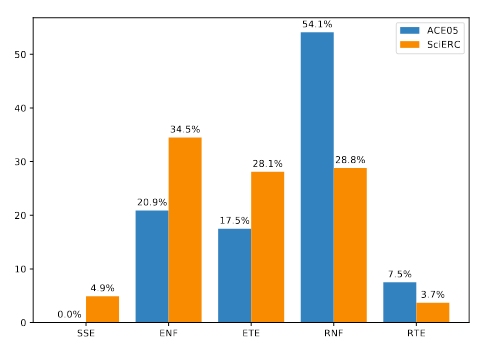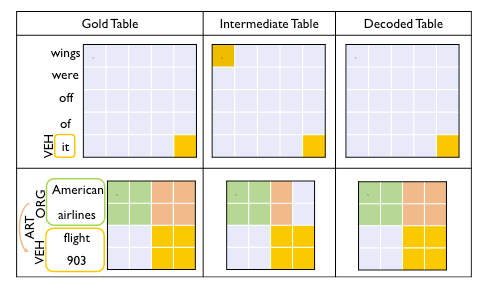## Summary

UniRE用一种统一标签, 尝试将ERE任务在一张实体关系统一表上用填表法解决, 理论上消除了实体空间和关系空间的偏差.

上一篇W2NER: Unified Named Entity Recognition as Word - Word Relation Classification
W2NER: Unified Named Entity Recognition as Word - Word Relation Classification本文是论文Unified Named Entity Recognition as W
2022-10-16DirectRel: Relational Triple Extraction - One Step is Enough
Relational Triple Extraction: One Step is Enough本文是论文Relational Triple Extraction: One Step is Enough 的阅读笔记和个人理解, 论文来自IJ
2022-08-31
目录